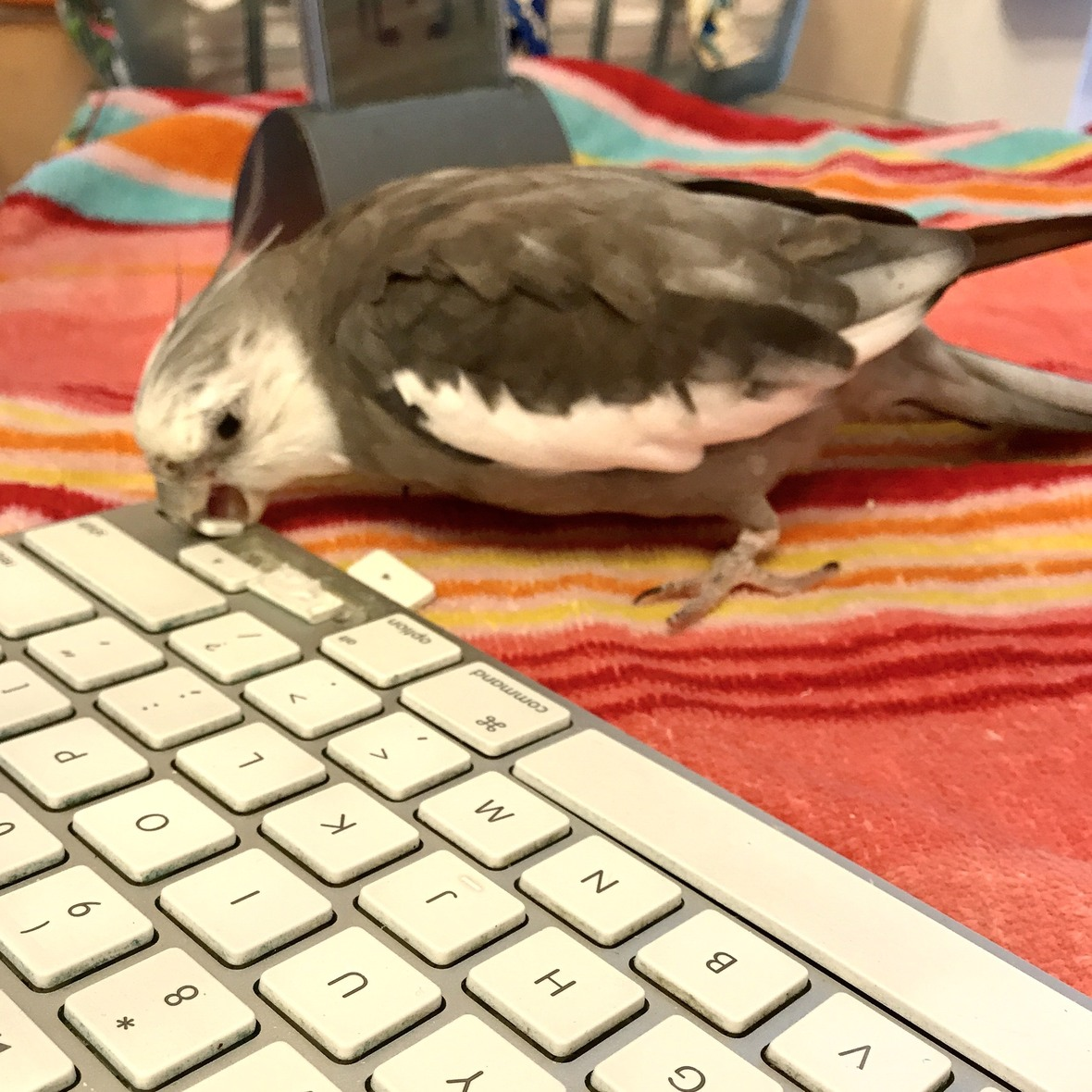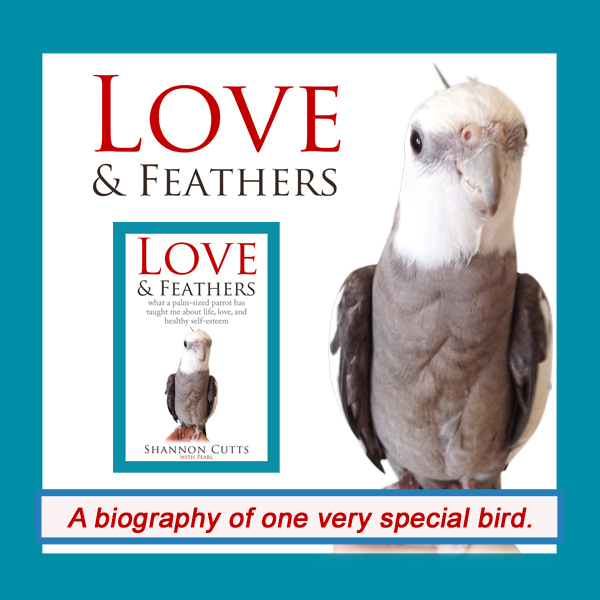# February 2019

 table div table+table+table div table{width:100%;padding:0}table div table+table+table div table img{width:96.23%;padding:0;float:none}table div table+table+table div table td{width:100%;padding:0 1.88% 18px}/* styles *//* styles */ Receiving this email as a forward? Sign up to keep following our little flock's adventures!
 table div table+table+table+table+table+table div table{width:100%;padding:0}table div table+table+table+table+table+table div table img{width:96.23%;padding:0;float:none}table div table+table+table+table+table+table div table td{width:100%;padding:0 1.88% 18px}/* styles */## What Do You REALLY Want?Pearl and his laptop keyboard keys - a pure heart desire that he doesn't question one bit!

For many years - decades, really - I have been striving towards a state I call not-wanting.

As many of you know, my initial aspirations were wildly misguided as I focused on not wanting food, calories, weight...the exact sort of not-wanting that can transform a reasonably healthy tween into a totally unhealthy anorexic teen.

Later, as I got better at this sort of not-wanting and my efforts made a sharp turn away from unhealthy and towards deadly, I decided to focus more specifically on not-wanting to die. Happily, this eventually resulted in recovery and a much healthier and more functional me.

Along the way, I discovered meditation. This led me once again to not-wanting, this time as a lifestyle choice in preparation for what many yogis and meditation masters call a "good death." After all, I reasoned, if I am going to die anyway and I only get one shot at it, I want to make sure it's a good one.

Why am I telling you all this? Basically, it is because I only just now realized that all the well-intentioned not-wanting in the world will never block out the pure and potent wanting that stretches down into the deepest fibers of my being.

I have also learned that this perpetual state of wanting is programmed right into our most fundamental hardwiring. For example, we all want food, water, shelter, to not get eaten. We all want connection. We all want to find our place in this world, whether it is our place in the pack or around the conference table. And we all want to fully be who we are - to fulfill our potential.

But only homo sapiens (us) have that large and pesky pre-frontal cortex to interfere with our deep knowledge and acceptance of what we want. Here, I mean what we REALLY want, not what our mind says we "should" want or what is reasonable to want or what we believe we are allowed to want or can want and actually get.

By the way, the difference between us and all other species in this area is HUGE.

I see it all the time with my trio. While I stew in a mental cesspool of indecision, continually second-guessing how I direct my energy, focus and ambition, Pearl heads straight for the crispy flingable off-limits laptop keyboard keys yet again, prying the keys off one by one and sending each one flying in a joyful skittering arc across the kitchen floor.

While I worry and wonder about whether it is right or reasonable to go for what I want, Malti reaches up and decisively rips off that huge cactus leaf, settling her increasingly sizable bulk down on top of one half of it while making a nice snack out of the other half.

As I attempt and then abort yet another creative writing project, arguing amongst myself about whether it has any intrinsic and thus worthwhile value to this world, Bruce clambers up the side of his wire mesh enclosure, heading for the freedom of the green, green grass and the lovely lady box turtle he is sure he will find waiting for him there.

To Pearl, Malti and Bruce, what they want is so simple, so innocent, so trustworthy, so clear. They don't doubt their fundamental desires. They don't waste a moment holding themselves back. They don't THINK their desires at all. They FEEL them, and that feeling is what sets them free to trust and act.

Not-wanting is a noble aspiration for sure. It is selfless and mature and surprisingly attainable. But it just isn't true, at least not for me.

Here is what IS true for me, in terms of what I truly, deeply want, in no particular order.
{Big, deep breath....GO!}

▪ I want to spend my life with the love of my life (you know who you are, even though I know you aren't quite there yet and may never be and probably aren't even reading this, thank goodness).
▪ I want to write that new book, the one that is digging and clawing its way up out of me even as I stuff it all back down inside and then sit down on it, HARD.
▪ I want to live tiny and minimalist because I want to love every single special and precious thing and being in my life, each as unique and unrepeatable as I myself am.
▪ I want to heal and stay healed (yes, thyroid, I am talking to you).
▪ I want to share my life forever with an interspecies flock that includes human beings and green beings and feathered beings and furred beings and shelled beings and all other beings (except mosquitos, thanks but no thanks).
▪ I want every day of my life to include meditation, yoga and coffee.
▪ I want to help - everything, everyone - by bringing a little more light, a little more laughter, a little more joy, a little more insight, a little more hope into our shared lives than was there before I was here.
 ▪ I want to spend my life with the love of my life (you know who you are, even though I know you aren't quite there yet and may never be and probably aren't even reading this, thank goodness).
 ▪ I want to write that new book, the one that is digging and clawing its way up out of me even as I stuff it all back down inside and then sit down on it, HARD.
 ▪ I want to live tiny and minimalist because I want to love every single special and precious thing and being in my life, each as unique and unrepeatable as I myself am.
 ▪ I want to heal and stay healed (yes, thyroid, I am talking to you).
 ▪ I want to share my life forever with an interspecies flock that includes human beings and green beings and feathered beings and furred beings and shelled beings and all other beings (except mosquitos, thanks but no thanks).
 ▪ I want every day of my life to include meditation, yoga and coffee.
 ▪ I want to help - everything, everyone - by bringing a little more light, a little more laughter, a little more joy, a little more insight, a little more hope into our shared lives than was there before I was here.

There is more. There are other wants, additional deep irrefutable, undeniable, pure and worthy wants where those came from. But these are the highlights.

Join me? What do you really, REALLY want?

with great love and respect,

Shannon

 table div table+table+table+table+table+table+table+table+table div table{width:100%;padding:0}table div table+table+table+table+table+table+table+table+table div table img{width:96.23%;padding:0;float:none}table div table+table+table+table+table+table+table+table+table div table td{width:100%;padding:0 1.88% 18px}/* styles *//* styles */ How do you figure out which wants are your "real" wants? Here, I am not talking about the wants you think you should want, or the wants you think you can actually get, or even the wants you think are mature or sufficiently selfless to want. How do you know what you really, truly, deeply want for yourself in this life? Do you ever struggle to let yourself want what you want - no permission, no explanation needed? I would love to hear your thoughts and stories!
 table div table+table+table+table+table+table+table+table+table+table+table div table{width:100%;padding:0}table div table+table+table+table+table+table+table+table+table+table+table div table img{width:96.23%;padding:0;float:none}table div table+table+table+table+table+table+table+table+table+table+table div table td{width:100%;padding:0 1.88% 18px}/* styles */# "Waffles & More" - Order Your Tasty Copy Today!

 table.module-12{width:75.47%;padding:0}table div table+table+table+table+table+table+table+table+table+table+table+table+table div table{width:75.47%;float:none;margin-left:auto;margin-right:auto;padding:0}table div table+table+table+table+table+table+table+table+table+table+table+table+table div table a{border:0 none;text-decoration:none}table div table+table+table+table+table+table+table+table+table+table+table+table+table div table img{width:100%!important;border:0 none;text-decoration:none}table div table+table+table+table+table+table+table+table+table+table+table+table+table div table td{width:100%;padding:0}/* styles */
 table div table+table+table+table+table+table+table+table+table+table+table+table+table+table+table div table{width:100%;padding:0}table div table+table+table+table+table+table+table+table+table+table+table+table+table+table+table div table img{width:96.23%;padding:0;float:none}table div table+table+table+table+table+table+table+table+table+table+table+table+table+table+table div table td{width:100%;padding:0 1.88% 18px}/* styles */# Get your copy of Love & Feathers the book!Click on the image of Pearl to order your signed copy!Pearl will even beak-o-graph it for you!
 table div table+table+table+table+table+table+table+table+table+table+table+table+table+table+table+table+table+table+table+table div table{width:100%;padding:0}table div table+table+table+table+table+table+table+table+table+table+table+table+table+table+table+table+table+table+table+table div table img{width:96.23%;padding:0;float:none}table div table+table+table+table+table+table+table+table+table+table+table+table+table+table+table+table+table+table+table+table div table td{width:100%;padding:0 1.88% 18px}/* styles */# Malti with her tasty cactus leaf.

 table.module-21{width:75.47%;padding:0}table div table+table+table+table+table+table+table+table+table+table+table+table+table+table+table+table+table+table+table+table+table+table div table{width:75.47%;float:none;margin-left:auto;margin-right:auto;padding:0}table div table+table+table+table+table+table+table+table+table+table+table+table+table+table+table+table+table+table+table+table+table+table div table a{border:0 none;text-decoration:none}table div table+table+table+table+table+table+table+table+table+table+table+table+table+table+table+table+table+table+table+table+table+table div table img{width:100%!important;border:0 none;text-decoration:none}table div table+table+table+table+table+table+table+table+table+table+table+table+table+table+table+table+table+table+table+table+table+table div table td{width:100%;padding:0}/* styles */
 table div table+table+table+table+table+table+table+table+table+table+table+table+table+table+table+table+table+table+table+table+table+table+table+table div table{width:100%;padding:0}table div table+table+table+table+table+table+table+table+table+table+table+table+table+table+table+table+table+table+table+table+table+table+table+table div table img{width:96.23%;padding:0;float:none}table div table+table+table+table+table+table+table+table+table+table+table+table+table+table+table+table+table+table+table+table+table+table+table+table div table td{width:100%;padding:0 1.88% 18px}/* styles */# Bruce is still hibernating! Sleepy boy!

 table.module-25{width:75.47%;padding:0}table div table+table+table+table+table+table+table+table+table+table+table+table+table+table+table+table+table+table+table+table+table+table+table+table+table+table div table{width:75.47%;float:none;margin-left:auto;margin-right:auto;padding:0}table div table+table+table+table+table+table+table+table+table+table+table+table+table+table+table+table+table+table+table+table+table+table+table+table+table+table div table a{border:0 none;text-decoration:none}table div table+table+table+table+table+table+table+table+table+table+table+table+table+table+table+table+table+table+table+table+table+table+table+table+table+table div table img{width:100%!important;border:0 none;text-decoration:none}table div table+table+table+table+table+table+table+table+table+table+table+table+table+table+table+table+table+table+table+table+table+table+table+table+table+table div table td{width:100%;padding:0}/* styles */
 table div table+table+table+table+table+table+table+table+table+table+table+table+table+table+table+table+table+table+table+table+table+table+table+table+table+table+table+table div table{width:100%;padding:0}table div table+table+table+table+table+table+table+table+table+table+table+table+table+table+table+table+table+table+table+table+table+table+table+table+table+table+table+table div table img{width:96.23%;padding:0;float:none}table div table+table+table+table+table+table+table+table+table+table+table+table+table+table+table+table+table+table+table+table+table+table+table+table+table+table+table+table div table td{width:100%;padding:0 1.88% 18px}/* styles */## Meet Pearl, Malti, Bruce & Me

 table div table+table+table+table+table+table+table+table+table+table+table+table+table+table+table+table+table+table+table+table+table+table+table+table+table+table+table+table+table+table div table,table.module-29{width:34.34%;float:left;padding:0}table div table+table+table+table+table+table+table+table+table+table+table+table+table+table+table+table+table+table+table+table+table+table+table+table+table+table+table+table+table+table div table a{border:0 none;text-decoration:none}table div table+table+table+table+table+table+table+table+table+table+table+table+table+table+table+table+table+table+table+table+table+table+table+table+table+table+table+table+table+table div table img{width:100%!important;border:0 none;text-decoration:none}table div table+table+table+table+table+table+table+table+table+table+table+table+table+table+table+table+table+table+table+table+table+table+table+table+table+table+table+table+table+table div table td{width:100%;padding:0 20px 20px 0}/* styles */ Hi! It is so good to meet you here each month! I'm so excited about our newest book, "Waffles & More: A Love & Feathers Recipe Book," which features so many of the recipes our flock (and especially the flock member with the feathers) particularly enjoys! And of course there is the book that started it all - "Love & Feathers: what a palm-sized parrot has taught me about life, love, and healthy self-esteem." This fun and feathery book focuses on the power of animal mentors in our lives and features my life with my precious parrot, Pearl. Malti is my darling young red-foot tortoise. She has such a healthy appetite for food, snoozing and life! Bruce, a very brave rescued Texas 3-toed box turtle, joined our flock a few years ago in a space clearly reserved just for him! My first book, "Beating Ana: how to outsmart your eating disorder and take your life back," is a practical hands-on workbook to help overcome eating disorders as well as the story of my personal recovery journey. MentorCONNECT, the first global eating disorders nonprofit mentoring community, emerged from the experience of writing "Beating Ana." While we closed in April 2017, the website continues to provide information about mentoring-based recovery support resources. ==> To connect with me: www.shannoncutts.com ==> To connect with Pearl, Malti & Bruce: www.loveandfeathersandshells.com
 table div table+table+table+table+table+table+table+table+table+table+table+table+table+table+table+table+table+table+table+table+table+table+table+table+table+table+table+table+table+table+table div table{width:100%;padding:0}table div table+table+table+table+table+table+table+table+table+table+table+table+table+table+table+table+table+table+table+table+table+table+table+table+table+table+table+table+table+table+table div table img{width:96.23%;padding:0;float:none}table div table+table+table+table+table+table+table+table+table+table+table+table+table+table+table+table+table+table+table+table+table+table+table+table+table+table+table+table+table+table+table div table td{width:100%;padding:0 1.88% 18px}/* styles */table div table+table+table+table+table+table+table+table+table+table+table+table+table+table+table+table+table+table+table+table+table+table+table+table+table+table+table+table+table+table+table+table div table td,table.module-31{width:100%;padding:0}table div table+table+table+table+table+table+table+table+table+table+table+table+table+table+table+table+table+table+table+table+table+table+table+table+table+table+table+table+table+table+table+table div table{width:100%;float:none;margin-left:auto;margin-right:auto;padding:0}table div table+table+table+table+table+table+table+table+table+table+table+table+table+table+table+table+table+table+table+table+table+table+table+table+table+table+table+table+table+table+table+table div table a{border:0 none;text-decoration:none}table div table+table+table+table+table+table+table+table+table+table+table+table+table+table+table+table+table+table+table+table+table+table+table+table+table+table+table+table+table+table+table+table div table img{width:100%!important;border:0 none;text-decoration:none}/* styles */
 table.module-32{width:74.15%;padding:0}table div table+table+table+table+table+table+table+table+table+table+table+table+table+table+table+table+table+table+table+table+table+table+table+table+table+table+table+table+table+table+table+table+table div table{width:74.15%;float:none;margin-left:auto;margin-right:auto;padding:0}table div table+table+table+table+table+table+table+table+table+table+table+table+table+table+table+table+table+table+table+table+table+table+table+table+table+table+table+table+table+table+table+table+table div table a{border:0 none;text-decoration:none}table div table+table+table+table+table+table+table+table+table+table+table+table+table+table+table+table+table+table+table+table+table+table+table+table+table+table+table+table+table+table+table+table+table div table img{width:100%!important;border:0 none;text-decoration:none}table div table+table+table+table+table+table+table+table+table+table+table+table+table+table+table+table+table+table+table+table+table+table+table+table+table+table+table+table+table+table+table+table+table div table td{width:100%;padding:0}/* styles */
 table div table+table+table+table+table+table+table+table+table+table+table+table+table+table+table+table+table+table+table+table+table+table+table+table+table+table+table+table+table+table+table+table+table+table div table{width:100%;padding:0}table div table+table+table+table+table+table+table+table+table+table+table+table+table+table+table+table+table+table+table+table+table+table+table+table+table+table+table+table+table+table+table+table+table+table div table img{width:96.23%;padding:0;float:none}table div table+table+table+table+table+table+table+table+table+table+table+table+table+table+table+table+table+table+table+table+table+table+table+table+table+table+table+table+table+table+table+table+table+table div table td{width:100%;padding:0 1.88% 18px}/* styles */## Reprints & Reposts

REPRINTS: Would you like to use any portion of this material in your newsletter, blog or website? You may do so as long as you also include this copyright information: